# 继承与派生

## 基本概念和语法

### 概念

• 继承与派生是同一过程从不同角度看
保持已有的特性而构造新类的过程称为继承。
在已有类的基础上新增自己的特性而产生新类的过程为派生。
• 被继承的已有类称为基类（父类）
• 派生出的新类称为派生类（或子类）
• 直接参与派生出某类的基类称为直接基类。
• 基类的基类甚至更高的基类称为间接基类。

### 定义

class 派生类名：继承方式 基类名{

}

class 派生类名：继承方式1 基类名,继承方式2 基类名2,...
{

}

• 吸收基类成员
• 改造基类的成员
• 添加新的成员

## 继承方式

• 派生类成员对基类成员的访问权限
• 通过派生类对象对基类成员的访问权限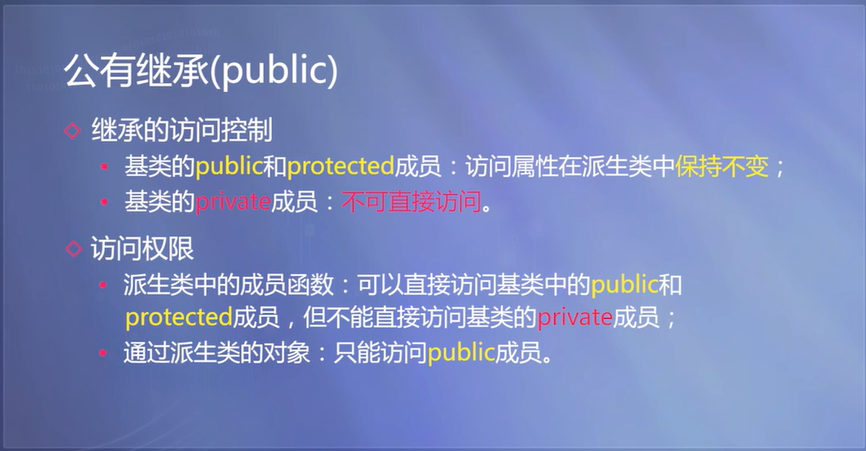using namespace std;

class Point{
public:
void initP(float xx,float yy)
{
x=xx;
y=yy;
}
void Move(float xOff,float yOff){
x+=xOff;
y+=yOff;
}
float GetX()const{return x;}
float GetY()const{return y;}
private:
float x,y;

};
class Rectangle:public Point{
public:
void initRectangle(float x,float y,float w,float h){
initP(x,y);
this->w=w;
this->h=h;
}
float GetH(){return h;}
float GetW(){return w;}
private:
float w,h;
};
int main(){
Rectangle a;
a.initRectangle(1,2,3,4);
a.Move(1,2);
cout<<a.GetX()<<endl;
cout<<a.GetY()<<endl;
cout<<a.GetW()<<endl;
cout<<a.GetH()<<endl;
return 0;
}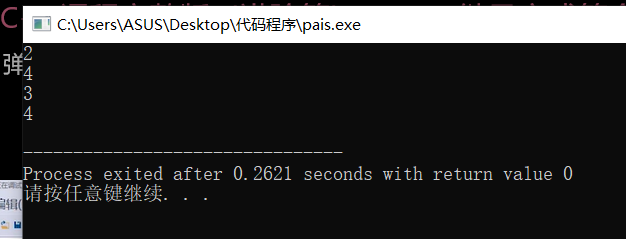## 私有继承和保护继承

• 私有继承：
私有继承，继承过来的父类的public只能在类内调用，但是不能被对象直接调用，私有继承的父类的公有成员为派生类的私有成员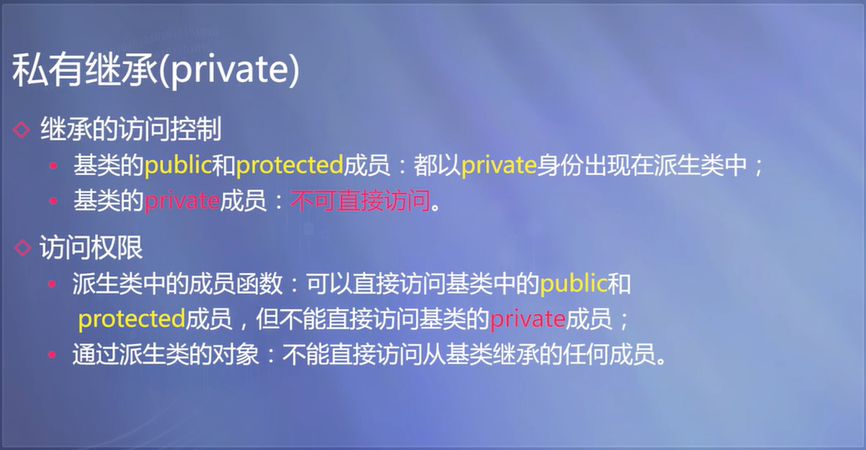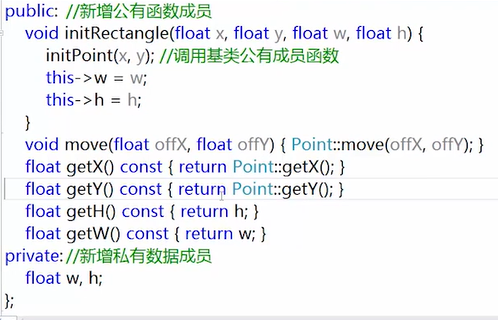• 保护继承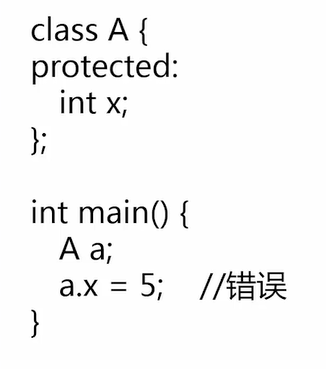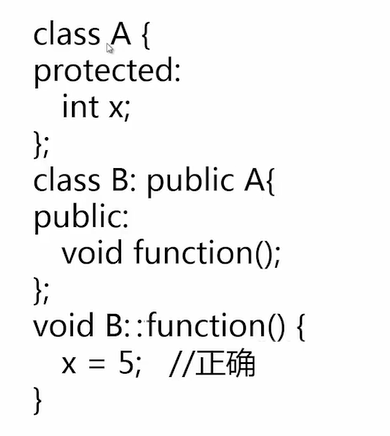#include<iostream>
using namespace std;
class A{
public:
void setA(int aa){
a = aa;
}
void showA()const{
cout<<a<<endl;
}
private:
int a;
};
class B{
public:
void setB(int bb){
b = bb;
}
void showB()const{
cout<<b<<endl;
}
private:
int b;
};
class C:public A,private B{
public:
void setC(int a,int b,int c){
setA(a);
setB(b);
this->c = c;
}
showC()const{
cout<<c<<endl;
}
private:
int c;
};
int main(){
C test;
test.setA(5);
test.showA();
test.setC(6,7,9);
test.showC();
test.setB(6);
}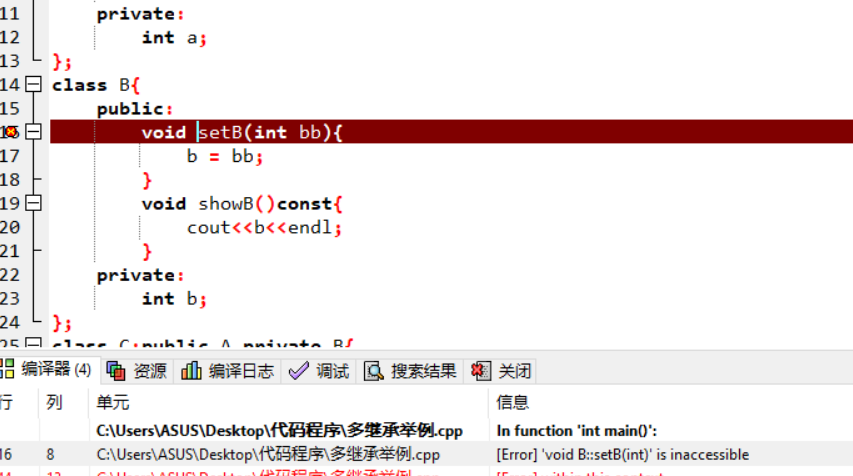posted @ 2019-10-13 17:17  醉死的乌鸦  阅读(161)  评论(0编辑  收藏  举报